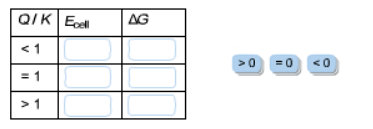# Problem: Complete this table relating the values of Ecell and ΔG to the Q/K ratio.

###### Problem Details

Complete this table relating the values of Ecell and ΔG to the Q/K ratio.What scientific concept do you need to know in order to solve this problem?

Our tutors have indicated that to solve this problem you will need to apply the The Nernst Equation concept. You can view video lessons to learn The Nernst Equation. Or if you need more The Nernst Equation practice, you can also practice The Nernst Equation practice problems.

What is the difficulty of this problem?

Our tutors rated the difficulty ofComplete this table relating the values of Ecell and ΔG to t...as medium difficulty.

How long does this problem take to solve?

Our expert Chemistry tutor, Dasha took 3 minutes and 34 seconds to solve this problem. You can follow their steps in the video explanation above.

What professor is this problem relevant for?

Based on our data, we think this problem is relevant for Professor Mohr's class at UIC.